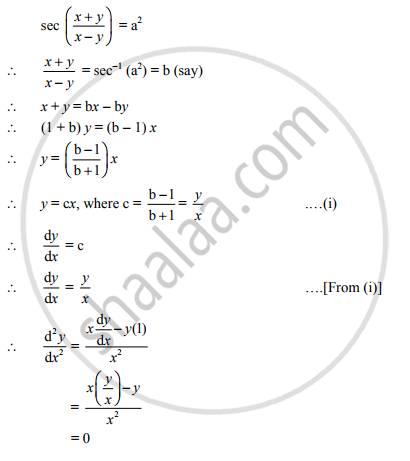# If sec((x+y)/(x-y))=a2. Then (d2y)/dx2= - Mathematics and Statistics

If sec((x+y)/(x-y))=a^2. " then " (d^2y)/dx^2=........

(a) y

(b) x

(c) y/x

(d) 0

#### Solution

(D) 0Concept: Derivatives of Inverse Trigonometric Functions
Is there an error in this question or solution?